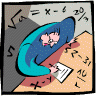## Mathematics, Department of## Dissertations, Theses, and Student Research Papers in Mathematics

Spring 4-21-2011

#### Comments

A DISSERTATION Presented to the Faculty of The Graduate College at the University of Nebraska In Partial Fulfilment of Requirements For the Degree of Doctor of Philosophy, Major: Mathematics, Under the Supervision of Professor Stephen G. Hartke. Lincoln, Nebraska: May, 2011
Copyright 2011 Tyler Seacrest

#### Abstract

This dissertation focuses on the intersection of two classical and fundamental areas in graph theory: graph packing and degree sequences. The question of packing degree sequences lies naturally in this intersection, asking when degree sequences have edge-disjoint realizations on the same vertex set. The most significant result in this area is Kundu's k-Factor Theorem, which characterizes when a degree sequence packs with a constant sequence. We prove a series of results in this spirit, and we particularly search for realizations of degree sequences with edge-disjoint 1-factors.

Perhaps the most fundamental result in degree sequence theory is the Erdos-Gallai Theorem, characterizing when a degree sequence has a realization. After exploring degree sequence packing, we develop several proofs of this famous theorem, connecting it to many other important graph theory concepts.

We are also interested in locating edge-disjoint 1-factors in dense graphs. Before tackling this question, we build on the work of Katerinis to find the largest k such that a graph has a k-factor, where the value of k depends on the minimum degree of the graph. This gives an upper bound on the number of edge-disjoint 1-factors.

The question of finding edge-disjoint 1-factors leads us to a conjecture of Bollobas and Scott about finding spanning balanced bipartite subgraphs with vertices of high degree. We first prove a degree-sequence version of the Bollobas--Scott Conjecture which we apply to the question of edge-disjoint 1-factors. We then generalize and prove an approximate version of the conjecture, yielding balanced partitions with many edges going to each part. This version has many applications, including finding edge-disjoint 1-factors and edge-disjoint Hamiltonian cycles.

Adviser: Stephen G. Hartke

COinS